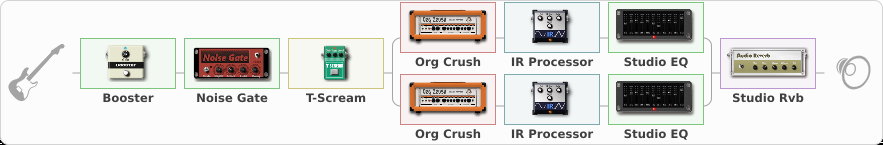# Djently Driven NOT !!

Discussion in 'ToneLib-GFX presets' started by Tetragramm1983, Dec 10, 2020.

1. ### Tetragramm1983Active Member

Djently Driven NOT !!
Preset name: Djently Driven NOT!

Tightly driven 7 String Djentiness. Drop A

omegaacolytes.bandcamp.com

Related video:

Effects chain:Effect: "Booster" (Dynamics / Filter), active - "yes"
{

"Gain" = 65
}

Effect: "Noise Gate" (Dynamics / Filter), active - "yes"
{

"Mode" = Manual
"Depth" = 100
"Threshold" = 20
"Attack" = 3
"Hold" = 11
"Decay" = 250
}

Effect: "T-Scream" (Overdrive / Distortion), active - "yes"
{

"Drive" = 10
"Tone" = 90
"Level" = 100
}

Effect: "Splitter" (Dynamics / Filter), active - "yes"
{

"A-Bypass" = Off
"A-Pan" = 0
"A-Level" = 55
"B-Bypass" = Off
"B-Pan" = 0
"B-Level" = 55
'A' branch:
{

Effect: "Org Crush" (Amp simulators), active - "yes"
{

"Gain" = 50
"Bass" = 35
"Middle" = 75
"Treble" = 81
"Presence" = 75
"Master" = 70
"Level (dB)" = 2
}
Effect: "IR Processor" (Cabinets), active - "yes"
{

"IR" = ML-MEGA-DJENT-MIX-DJENT
"Low Cut (Hz)" = 186
"Hi Cut (kHz)" = 16.5
"Mix" = 100
"Level (dB)" = 8
}
Effect: "Studio EQ" (Dynamics / Filter), active - "yes"
{

"31 Hz" = -4
"62 Hz" = -4
"125 Hz" = -6
"250 Hz" = -2
"500 Hz" = -6
"1 kHz" = -10
"2 kHz" = 2
"4 kHz" = -6
"8 kHz" = 4
"16 kHz" = 6
"Level (dB)" = 0
}
}
'B' branch:
{

Effect: "Org Crush" (Amp simulators), active - "yes"
{

"Gain" = 50
"Bass" = 45
"Middle" = 25
"Treble" = 65
"Presence" = 75
"Master" = 70
"Level (dB)" = 2
}
Effect: "IR Processor" (Cabinets), active - "yes"
{

"IR" = ML-MEGA-DJENT-MIX-GRIND
"Low Cut (Hz)" = 186
"Hi Cut (kHz)" = 16.5
"Mix" = 100
"Level (dB)" = 8
}
Effect: "Studio EQ" (Dynamics / Filter), active - "yes"
{

"31 Hz" = -6
"62 Hz" = -6
"125 Hz" = -6
"250 Hz" = -4
"500 Hz" = -10
"1 kHz" = 2
"2 kHz" = -4
"4 kHz" = -4
"8 kHz" = 4
"16 kHz" = 4
"Level (dB)" = 0
}
}
}

Effect: "Studio Rvb" (Reverberation), active - "yes"
{

"Time" = 3.9
"PreDelay" = 8
"LoDamp" = 0
"HiDamp" = 10
"Mix" = 35
}

Note: You will need to download and install the ToneLib-GFX software to use the preset.

File size:
25.1 KB
Views:
2,435